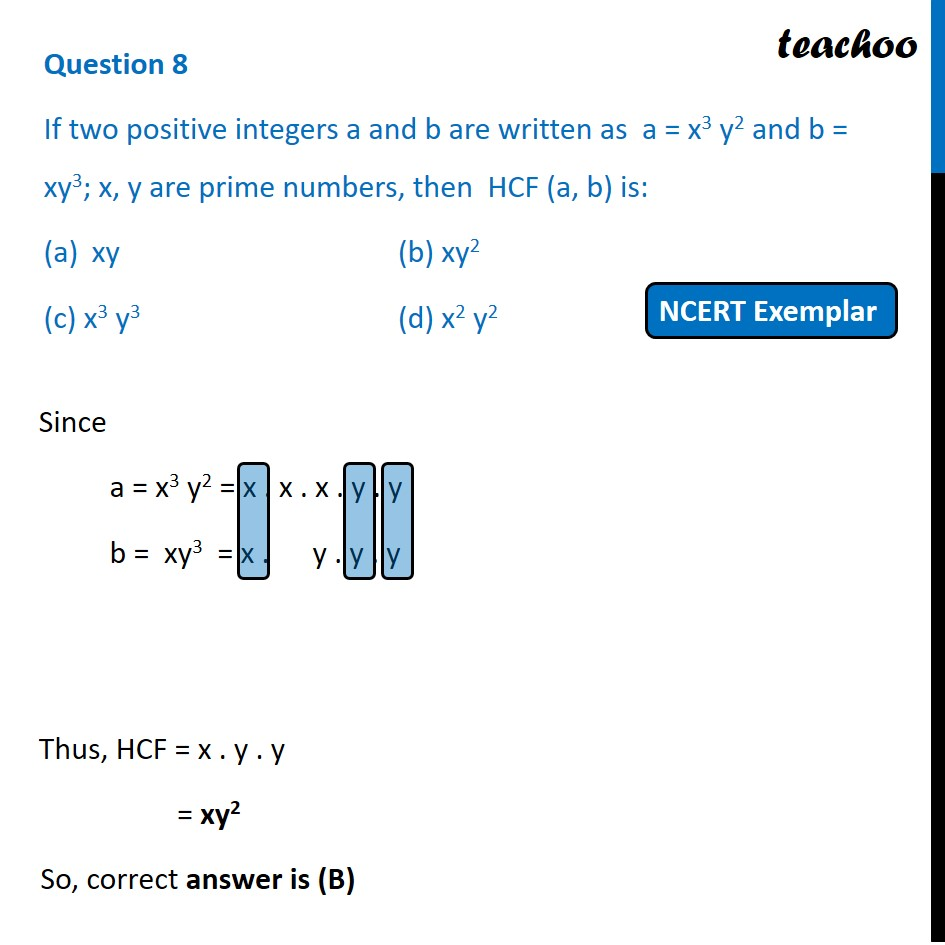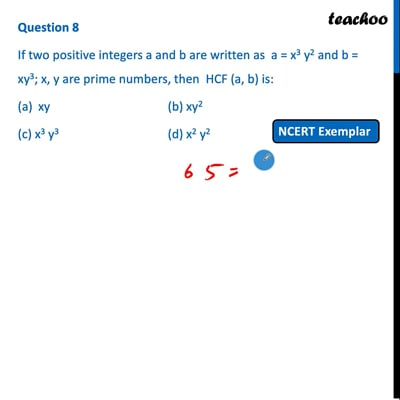MCQs from NCERT Exemplar

Chapter 1 Class 10 Real Numbers
Serial order wise

## (c) x 3 y 3                    (d) x2 y 2This video is only available for Teachoo black users

Introducing your new favourite teacher - Teachoo Black, at only ₹83 per month

### Transcript

Question 8 If two positive integers a and b are written as a = x3 y2 and b = xy3; x, y are prime numbers, then HCF (a, b) is: xy (b) xy2 (c) x3 y3 (d) x2 y2 Since a = x3 y2 = x . x . x . y . y b = xy3 = x . y . y . y Thus, HCF = x . y . y = xy2 So, correct answer is (B)